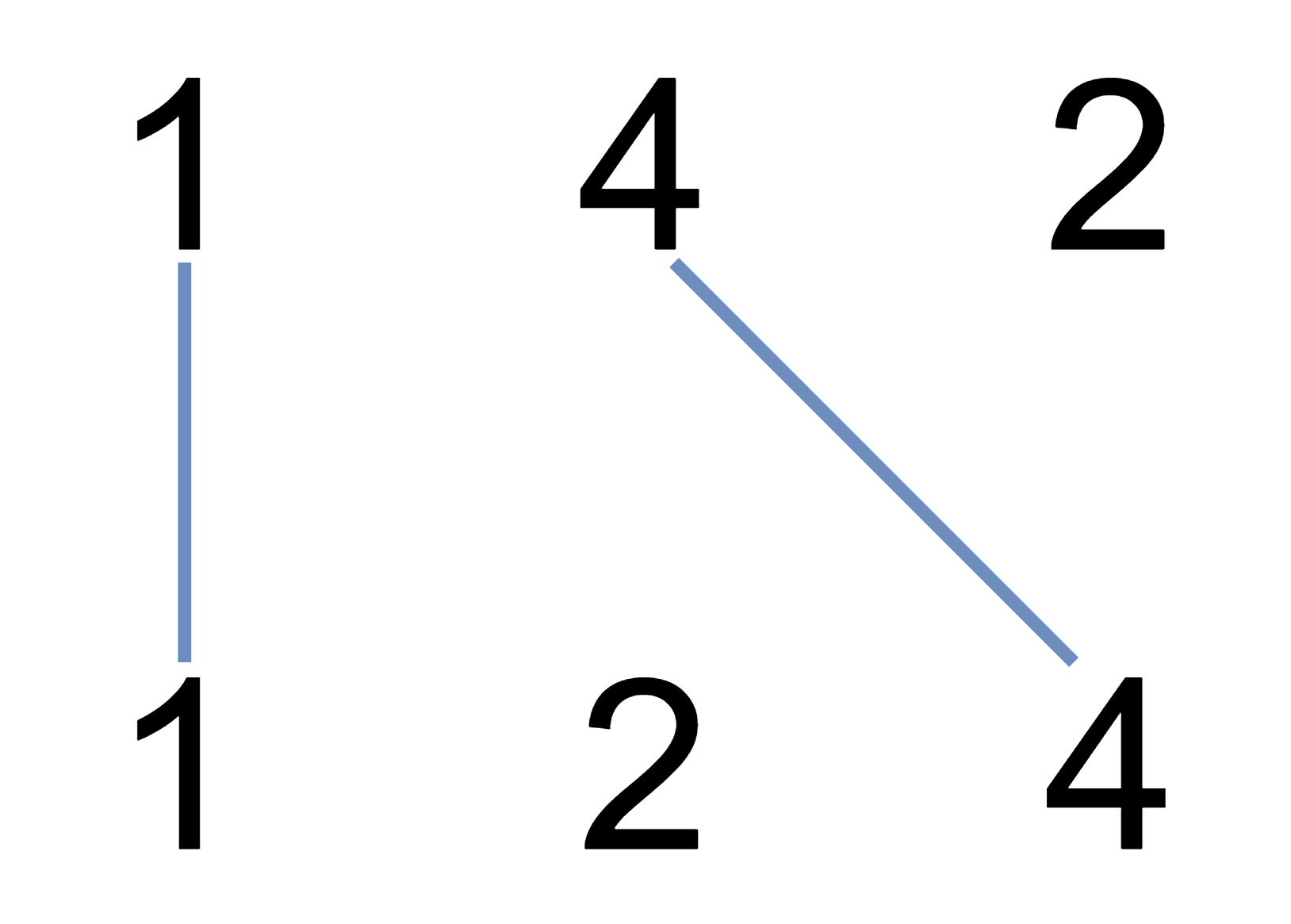##### Welcome to Subscribe On Youtube

Formatted question description: https://leetcode.ca/all/1035.html

# 1035. Uncrossed Lines (Medium)

We write the integers of A and B (in the order they are given) on two separate horizontal lines.

Now, we may draw connecting lines: a straight line connecting two numbers A[i] and B[j] such that:

• A[i] == B[j];
• The line we draw does not intersect any other connecting (non-horizontal) line.

Note that a connecting lines cannot intersect even at the endpoints: each number can only belong to one connecting line.

Return the maximum number of connecting lines we can draw in this way.

Example 1:Input: A = [1,4,2], B = [1,2,4]
Output: 2
Explanation: We can draw 2 uncrossed lines as in the diagram.
We cannot draw 3 uncrossed lines, because the line from A=4 to B=4 will intersect the line from A=2 to B=2.


Example 2:

Input: A = [2,5,1,2,5], B = [10,5,2,1,5,2]
Output: 3


Example 3:

Input: A = [1,3,7,1,7,5], B = [1,9,2,5,1]
Output: 2

Note:

1. 1 <= A.length <= 500
2. 1 <= B.length <= 500
3. 1 <= A[i], B[i] <= 2000

Related Topics:
Array

Similar Questions:

## Solution 1. DP

This problem is equivalent to longest common subsequence.

Let dp[i + 1][j + 1] be the maximum number of connecting lines between A[0..i] and B[0..j].

dp[i+1][j+1] = max( dp[i+1][j], dp[i][j+1] )         if A[i] != B[j]
1 + dp[i][j]                          if A[i] == B[j]
dp = 0

// OJ: https://leetcode.com/problems/uncrossed-lines/
// Time: O(MN)
// Space: O(MN)
class Solution {
public:
int maxUncrossedLines(vector<int>& A, vector<int>& B) {
int M = A.size(), N = B.size();
vector<vector<int>> dp(M + 1, vector<int>(N + 1));
for (int i = 0; i < M; ++i) {
for (int j = 0; j < N; ++j) {
if (A[i] == B[j]) dp[i + 1][j + 1] = 1 + dp[i][j];
else dp[i + 1][j + 1] = max(dp[i][j + 1], dp[i + 1][j]);
}
}
return dp[M][N];
}
};


## Solution 2. DP + Space Optimization

// OJ: https://leetcode.com/problems/uncrossed-lines/
// Time: O(MN)
// Space: O(min(M, N))
class Solution {
public:
int maxUncrossedLines(vector<int>& A, vector<int>& B) {
int M = A.size(), N = B.size();
if (M < N) swap(M, N), swap(A, B);
vector<int> dp(N + 1);
for (int i = 0; i < M; ++i) {
int prev = 0;
for (int j = 0; j < N; ++j) {
int cur = dp[j + 1];
if (A[i] == B[j]) dp[j + 1] = 1 + prev;
else dp[j + 1] = max(dp[j + 1], dp[j]);
prev = cur;
}
}
return dp[N];
}
};


Java

• class Solution {
public int maxUncrossedLines(int[] A, int[] B) {
int length1 = A.length, length2 = B.length;
int[][] dp = new int[length1 + 1][length2 + 1];
for (int i = 1; i <= length1; i++) {
int num1 = A[i - 1];
for (int j = 1; j <= length2; j++) {
int num2 = B[j - 1];
if (num1 == num2)
dp[i][j] = dp[i - 1][j - 1] + 1;
else
dp[i][j] = Math.max(dp[i - 1][j], dp[i][j - 1]);
}
}
return dp[length1][length2];
}
}

• // OJ: https://leetcode.com/problems/uncrossed-lines/
// Time: O(MN)
// Space: O(MN)
class Solution {
public:
int maxUncrossedLines(vector<int>& A, vector<int>& B) {
int M = A.size(), N = B.size();
vector<vector<int>> dp(M + 1, vector<int>(N + 1));
for (int i = 0; i < M; ++i) {
for (int j = 0; j < N; ++j) {
if (A[i] == B[j]) dp[i + 1][j + 1] = 1 + dp[i][j];
else dp[i + 1][j + 1] = max(dp[i][j + 1], dp[i + 1][j]);
}
}
return dp[M][N];
}
};

• class Solution:
def maxUncrossedLines(self, nums1: List[int], nums2: List[int]) -> int:
m, n = len(nums1), len(nums2)
dp = [ * (n + 1) for i in range(m + 1)]
for i in range(1, m + 1):
for j in range(1, n + 1):
if nums1[i - 1] == nums2[j - 1]:
dp[i][j] = dp[i - 1][j - 1] + 1
else:
dp[i][j] = max(dp[i - 1][j], dp[i][j - 1])
return dp[m][n]

############

# 1035. Uncrossed Lines
# https://leetcode.com/problems/uncrossed-lines/

class Solution:
def maxUncrossedLines(self, nums1: List[int], nums2: List[int]) -> int:
m, n = len(nums1), len(nums2)
dp = [ * (n + 1) for _ in range(m + 1)]

for i in range(m):
for j in range(n):
if nums1[i] == nums2[j]:
dp[i + 1][j + 1] = 1 + dp[i][j]
else:
dp[i + 1][j + 1] = max(dp[i + 1][j], dp[i][j + 1])

return dp[m][n]